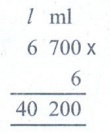Home | | Maths 5th Std | Exercise 4.2 (capacity)

# Exercise 4.2 (capacity)

Measurements : Conversion, Addition, Subtraction, Multiplication and Division in capacity

Exercise 4.2

1. Fill in the blanks

(i) Millilitre is the smallest metric measure of capacity.

(ii) Kilolitre is the largest unit of volume and equals 1000 litres.

(iii) 7 kl 30 l = 7030 l.

(iv) 5 l 400 ml = 5400 ml.

(v) 1300 ml = 1 l 300 ml

2. Match the following

(i) 4500 ml – 6l 500 ml

(ii) 3250 ml − 8 l 200 ml

(iii) 6500 ml − 7 l 50 ml

(iv) 8200 ml − 4 l 500 ml

(v) 7050 ml − 3 l 250 ml

(i) 4500 ml − 4 l 500 ml

(ii) 3250 ml − 3 l 250 ml

(iii) 6500 ml − 6 l 500 ml

(iv) 8200 ml − 8 l 200 ml

(v) 7050 ml − 7 l 50 ml

3. Add and write in litres

(i) 400 l; 50 l; 500 ml

400 l + 50 l + 500 ml = 450 l 500 ml

(ii) 3 kl; 400 l; 3 ml

3000 l + 400 l + 3 ml = 3400 l 3 ml

(iii) 1400 ml; 5680 ml; 280 l

1 l 400 ml + 5 l 680 ml + 280 l = 287 l 080ml

4. Subtract:

(i) 3 kl from 15485 l

15485 l15 kl 485 l (−)12kl 485 l

15485 l − 3kl = 12 kl 485 l

(ii) 15 kl from 20 kl20 kl −15 kl = 5kl

(iii) 345 ml from 5l5 l – 345 ml = 4 l 655 ml

5. Multiply the following:

(i) 3 l 200 ml × 83 l 200 ml × 8 = 25 l 600 ml

(ii) 4 l 450 ml × 44 l 450 ml × 4 = 17 l 800 ml

(iii) 5 l 300 ml × 55 l 300 ml × 5 = 26 l 500 ml

(iv) 6 l 700 ml × 66 l 700 ml × 6 = 40 l 200 ml

6. Divide the following:

(i) 18 l 240 ml ÷ 618 l 240 ml ÷ 6 = 3 l 040 ml

(ii) 20 l 600 ml ÷ 220 l 600 ml ÷ 2 = 10 l 300 ml

(iii) 21 l 490 ml ÷ 721 l 490 ml ÷ 7 = 3 l 070 ml

(iv) 25 l 350 ml ÷ 525 l 350 ml ÷ 5 = 5 l 070 ml

7. Kalaiyarasi bought 5 l 500 ml groundnut oil and 750 ml sesame oil. How much oil did she bought in all?Oil she bought in all = 6 l 250ml

8. In a fuel station there was 70 l 500 ml of fuel. How much amount of fuel will be left after selling 35 l 700 ml of fuel?Fuel left after selling = 34 l 800ml

9. A pot contains 9 l 500 ml of water, how much amount of water will 7 such pots contain?

Amount of water contain in a pot = 9 l 500 ml

Amount of water contain in 7 pots =Amount of water contain in 7 pots = 66 l 500ml

10. 25 l 500 ml of milk is filled in 5 milk cans, how much milk is filled in one can?

Milk filled in 5 milk cans = 25 l 500ml

Milk filled in one milk can = 25l 500ml ÷ 5Milk filled in one milk can = 5 l 100 ml

Exercise 4.2

1. i) Millilitre ii) Kilolitre, 1000 iii) 7030 l iv) 5400ml v) 1l 300ml

2. (i) 4500 ml − 4 l 500 ml

(ii) 3250 ml − 3 l 250 ml

(ii 6500 ml − 6 l 500 ml

(iv) 8200 ml − 8 l 200 ml

(v) 7050 ml − 7 l 50 ml

3. i) 450l 500ml ii) 3400l 3ml iii) 287l 080ml

4. i) 12485l ii) 5kl iii) 4l 655ml

5. i) 25l 600ml ii) 17l 800ml iii) 26l 500ml iv) 40l 200ml

6. i) 3l 40ml ii) 10l 300ml iii) 3l 70ml iv) 5l 70ml

7. 6l 250ml 8. 34l 800ml

9. 66l 500ml

10. 5l 100ml

Tags : Measurements | Term 2 Chapter 4 | 5th Maths , 5th Maths : Term 2 Unit 4 : Measurements
Study Material, Lecturing Notes, Assignment, Reference, Wiki description explanation, brief detail
5th Maths : Term 2 Unit 4 : Measurements : Exercise 4.2 (capacity) | Measurements | Term 2 Chapter 4 | 5th Maths